# Stein space

(diff) ← Older revision | Latest revision (diff) | Newer revision → (diff)

holomorphically-complete space

A paracompact complex analytic space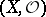with the following properties:

1) any compact analytic subset inis finite (cf. Analytic set 6));

2) any compact set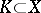has an open neighbourhoodinsuch thatis compact (weak holomorphic convexity).

A complex manifoldis a Stein space if and only ifis a Stein manifold. A complex space is a Stein space if and only if its reduction has this property. Any holomorphically-convex open subspace in a Stein space is a Stein space. A reduced complex space is a Stein space if and only if its normalization is a Stein space. Any closed analytic subspace in a Stein space, for instance in, is a Stein space. Any finite-dimensional Stein space has a proper injective holomorphic mapping (cf. Proper morphism) into somewhich is regular at every non-singular point. Any unramified covering of a Stein space is a Stein space. The direct product of two Stein spaces is a Stein space. In many cases a holomorphic fibre space whose base and fibres are Stein spaces is a Stein space (e.g. if the structure group is a complex Lie group with a finite number of connected components). However, there are holomorphic fibre spaces with fibre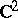and basethat are not Stein manifolds .

Let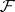be a coherent analytic sheaf on a Stein space. Then the following theoremsandof H. Cartan (cf. Cartan theorem) hold:

A) The space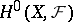generates the stalkof the sheafat any point;

B)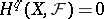for all.

Conversely, if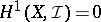for any coherent sheaf of ideals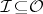, thenis a Stein space. A domain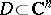is a Stein manifold if and only if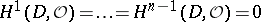.

From the Cartan theorems it follows that on a Stein space the first Cousin problem is always solvable, and if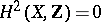, then the second Cousin problem is solvable as well (see Cousin problems). On any Stein manifoldthe Poincaré problem, i.e. can any meromorphic function be represented in the form, where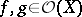,, is solvable. Furthermore, if, thenandcan be chosen in such a way that the germsat any pointare relatively prime. The group of divisor classes of an irreducible reduced Stein spaceis isomorphic to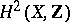. For any-dimensional Stein space, the homology groupsfor, and the group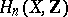is torsion-free. Ifis a manifold, thenis homotopy equivalent to an-dimensional cell complex. On the other hand, for any countable Abelian groupand anythere is a domain of holomorphy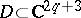such that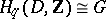.

An important trend in the theory of Stein spaces is connected with studies of the plurisubharmonic functions on them (see Levi problem; Pseudo-convex and pseudo-concave). The basic result here is that a Stein space is characterized as a space on which there exists a strongly-pseudoconvex function exhausting it.

Algebras of holomorphic functionson a Stein space(so-called Stein algebras) have the following properties. For a maximal ideal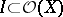the following conditions are equivalent:is closed in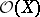with respect to the topology of compact convergence;for some point; andis finitely generated. Ifis finite-dimensional, then each character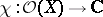is of the form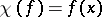for some. If,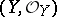are two finite-dimensional Stein spaces with isomorphic algebras, then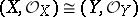; moreover, any isomorphism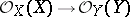is continuous and is induced by some isomorphismof complex spaces.

A significant role in the theory of Stein spaces is played by the so-called Oka principle, which states that a problem in the class of analytic functions on a Stein space is solvable if and only if it is solvable in the class of continuous functions. The second Cousin problem satisfies this principle. The following statement is still more general: The classification of the principal analytic fibrations (cf. Principal analytic fibration) with as basis a given reduced Stein spaceand as structure group a given complex Lie group, coincides with the classification of the topological fibrations with the same basis and the same structure group. The groups of connected components in the groups of analytic and continuous functionsalso coincide.

How to Cite This Entry:
Stein space. Encyclopedia of Mathematics. URL: http://encyclopediaofmath.org/index.php?title=Stein_space&oldid=17413
This article was adapted from an original article by A.L. Onishchik (originator), which appeared in Encyclopedia of Mathematics - ISBN 1402006098. See original article